# Dice Math III – Summing dice

It’s been a while since the last time (part I and part II). Besides dice pools and linear RNGs, a common way to get a random number is to roll a few dice and sum their results. This results in a curved distribution where some results are more probable than others. For example, rolling 3d6, you can only get result 3 by getting three ones but there are multiple ways of adding to e.g. 7 using numbers between 1 and 6.

A few possible probability distributions are shown in the next figure.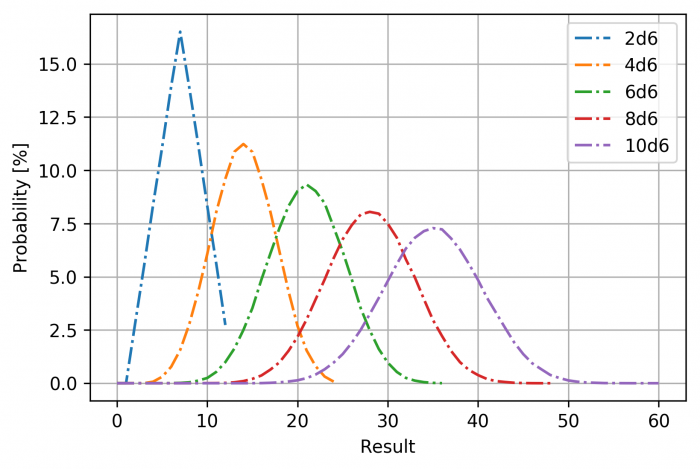It is immediately obvious that extreme values are increasingly unlikely as the number of dice goes up. In practice, resolution mechanics rarely use a very large number of dice, but damage in D&D is often determined by throwing a handful of dice – sneak attack and fireball, for example.

Astute readers might have already noticed that the distributions actually resemble ones produced by dicepools quite a lot and there is a good reason for that. And that is central limit theorem. This means that for large number of dice you can approximate the system using a gaussian distribution. However, this is often far more mathematically involving than is necessary and in the the context of pen and paper RPGs, brute force simulations are often the most straightforward way to design a system and indeed, that is what we shall do in this post, but we can take a couple of analytical results and apply them to this situation.

Just for completeness, here is the cumulative probability distribution.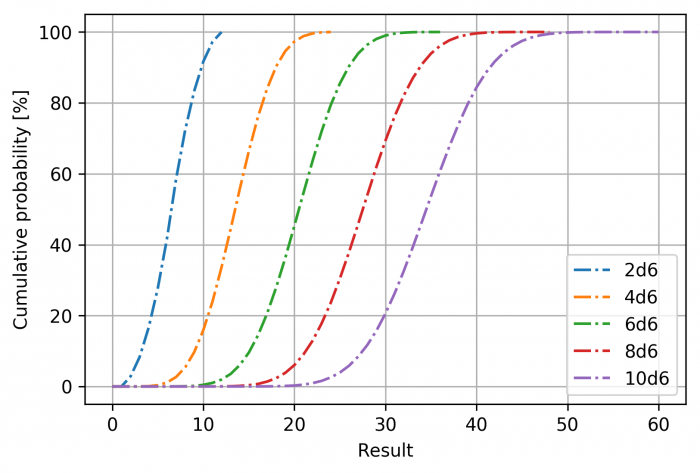The graph is read such that there is for example about 85% chance that the resulting sum for 10 d6s is 40 or lower.

It seems that the sum-RNG has much of the same qualitative properties as the dice pool mechanic. Let’s then take a closer look to a system where success is determined by 3d6 + bonus. First, here is the probability distribution of the specific RNG.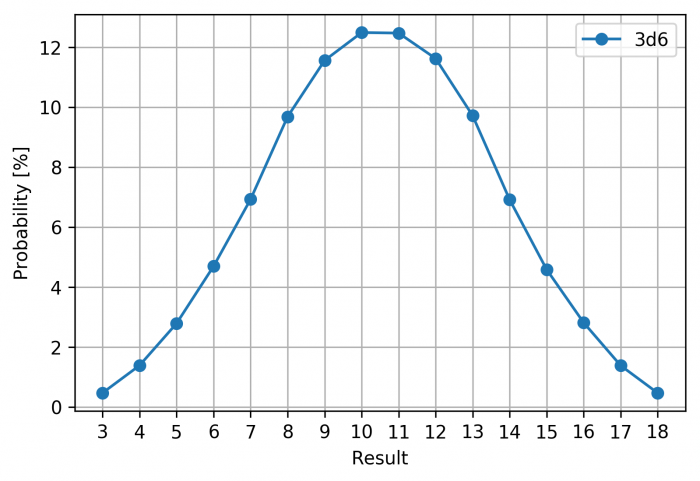And then the cumulative distribution.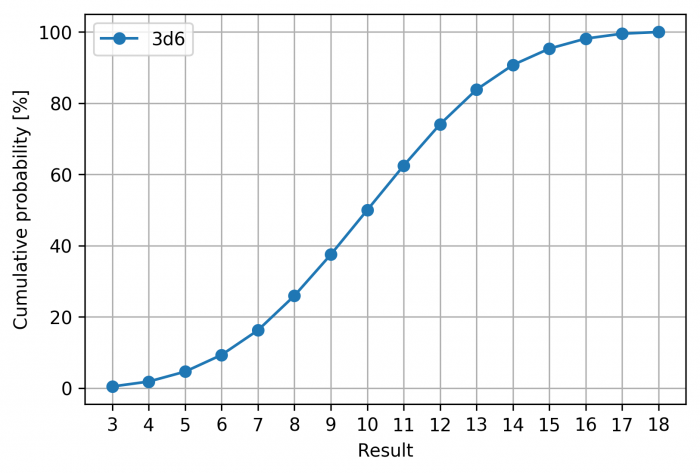The cumulative distribution is read such that there is for example around 40% probability of getting a result 9 or lower, i.e. around 60% of time, you will get a result higher than 9.

Sometimes 3d6 is presented as a replacement for a d20 if you want to get a little different probability distribution. However, 3d6 has only 16 distinct outcomes but it’s mean is 10.5 i.e. the same as with a d20. But as you recall, each result of a d20 has the same probability so the effects in game will be a little different. To illustrate these differences, consider opposed test – which we wanted to do anyways. The following is a graph of opposed test with the difference in bonuses going from -17 to +17. I have included a situation with 3d6 + modifier and d20 + modifiers.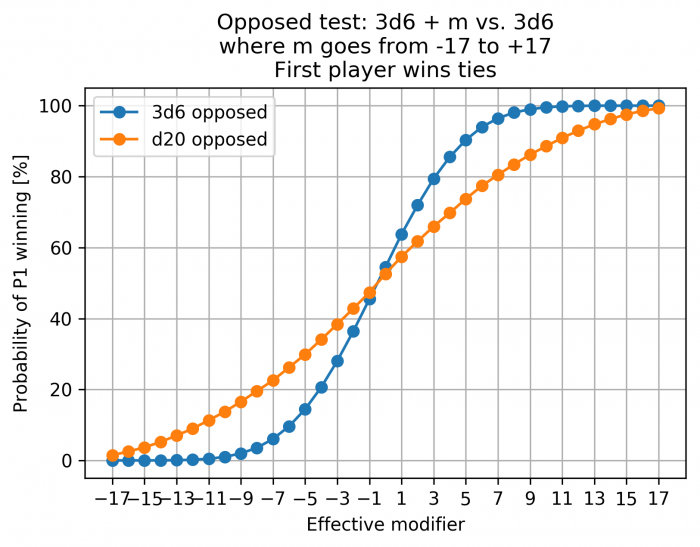As you can see, differences in modifiers result into much more one sided conflicts if you use a 3d6 as the random component. Even as small difference as -3 already means that the first player wins only 30% of time as opposed to roughly 40% of time for a d20. This is of course not to say that such a difference is bad, but rather that it is something to keep in mind.

These examples were for 3d6, but they are very easy to reproduce for other numbers. I’ll try to clean up my scripts to perhaps upload them here, but to be frank, if you can get python running, you probably can also create similar scipts by yourself.

Finally, there are a few possible modifications. One is rollign more dice than necessary and removing the lowest. Another is rerolling ones. The first system is commonly used for rolling stats in D&D 3rd edition, i.e. roll 4d6 and sum the three highest numbers but such RNG could also be used as a general resolution mechanic. Below, I have plotted the distribution of ‘4d6 pick 3 best’ vs 3d6.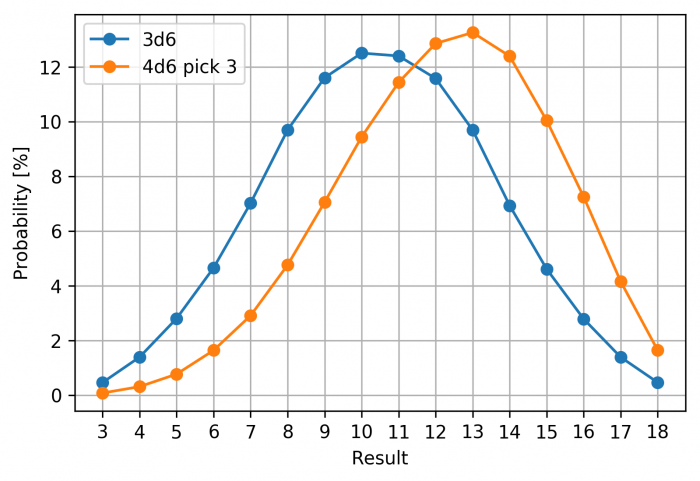As expected, the distribution shifts towards higher results and is no longer symmetric. You can read the differences most easily from the cumulative distribution.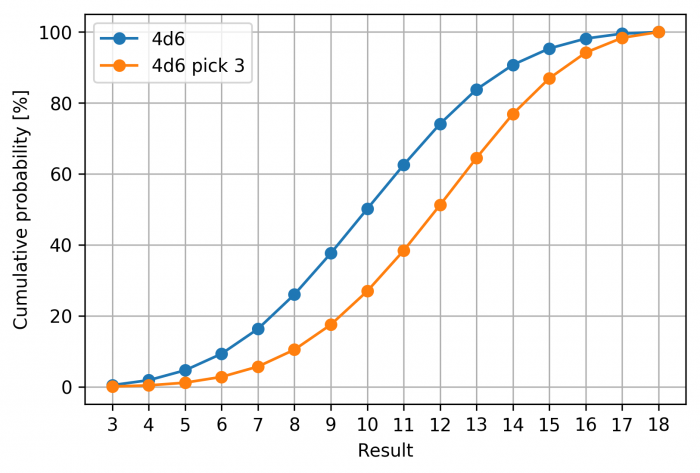Recall that the percentage is the probability of getting that number or lower. So 40 percent of time with 4d6 pick 3, you will get 11 or lower.

If I’ll figure other common modifications or situations that weren’t covered, I might do a short addendum later, but simulating such a system is nothing special.

Let’s finish by doing a little wrap up of the thing we have learned.

• Qualitatively similar to a dice pool -system, i.e. it can offer many of the same properties.
• Resolution time likely goes up quickly with the size of the ‘pool’. Adding a couple of numbers together is still quite fast, but doing so for e.g. 10 dice is already taxing.
• The number of dice thrown stays reasonable compared to a dice pool.
• The effect of a bonus is nontrivial. This also happens with dicepools, but at leas there one die is equals P successes, where P is the probability to score a hit with one die. Of course it’s not easy to estimate the probability of getting 6 or more hits with dicepool sizes 10 vs 11. However, for a small sum-RNG, a table can easily be prepared for probabilities of getting X or better result – in fact, this information is provided for fudge dice in the packaging – or you can just read the required information from the cumulative distribution. For dicepools, such a graph (or table) would need to be produced for every dice pool size. Of course the same information could be calculated in game with a simple app or script, but that might be a bit of a faux pas.
• Automatic wins are possible with static bonuses – and let’s be frank, static bonuses are practically always present in a ‘sum-RNG’ system.
• Advantage in opposed tests is massive for even small differences in bonuses

So in the end, probability-wise, a NdX + bonus might be easier to grasp for players and DMs, but before designing a game around it, think about the alternatives and peculiarities of this particular mechanic.